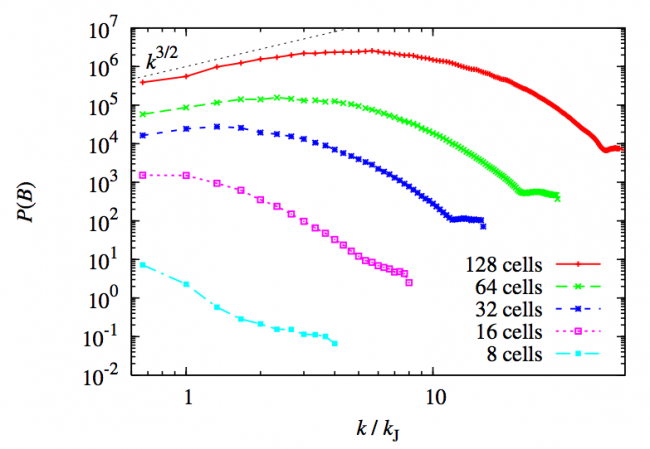# Magnetic Fields and Gravitational Collapse

A professor once told me that the key to doing astrophysics is to understand what physics is important in a given problem.  Can radiative transfer and cooling be ignored?  Are relativistic effects relevant?  These and many other questions are often best answered by considering the typical time/size/energy scales of a problem, and then deciding what physical processes are important at those scales.

It has become apparent in recent years that in the gravitational collapse of gas clouds (a process which can lead, for example, to star formation), magnetic fields may play a crucial role.  Even with an extremely small initial field (or “seed”), the compression of the gas and small-scale dynamo action can lead to a greatly amplified field, by many orders of magnitude.  One major way that the field gets enhanced is simply through the collapse of the gas; since astrophysical gas tends to carry the magnetic field lines along with it—the so-called “frozen flux approximation”, valid in the case of low resistivity and high ionization— so that the collapsed cloud has a much higher magnetic pressure than the initial, diffuse cloud.  Another important mechanism of magnetic field amplification is dynamo effects, where, on smaller scales, astrophysical turbulence can fold and tangle the field lines (see Brandenburg & Subramanian 2005 for an extensive review of this phenomenon).  Also, see the picture for an idea of how tangled field lines can get in a collapsed gas cloud.

In order to get a good handle on the role of magnetic fields in gas collapse scenarios, it is often necessary to run computer simulations.  The reason for this is that the combination of self-gravity, turbulence, and magnetic fields creates a 3-D, highly non-linear system that is often difficult to capture in a purely analytic calculation.  In light of this, the authors use magnetohydrodynamic (MHD) simulations to explore the dynamics of magnetic fields in collapsing gas clouds.

## Gravitational Collapse: Important Scales

Let us back up a little bit.  At the beginning of this astrobite, I briefly mentioned the importance of getting a handle on relevant scales of the problem.  For a collapsing gas cloud, one relevant time-scale is the “free-fall time”, or the characteristic time that it takes something to collapse under its own self-gravity, assuming no other forces resist the collapse.  The free-fall time$t_{ff}$ is proportional to$rho^{-1/2}$ where$rho$ denotes the density of the object (interestingly enough,$t_{ff}$ is independent of the actual size of the object).  Another time-scale which is often important, especially in the context of star formation, is the Kelvin-Helmholtz timescale, which gives an idea of how long a cloud of a given luminosity would need to collapse, if it lost all of its gravitational potential energy by radiating it away. An important mass scale is set by the Jeans mass, which has been touched on in past astrobites.  This is the mass of a cloud beyond which gravitational attraction will overcome the stabilizing effect of the internal gas pressure, and the cloud will collapse.  The related Jeans length$lambda_J$ is the size of such a cloud (so clouds of the same density that have size$lambda > lambda_J$ will collapse).

## Simulating Gas Collapse

We’ll now return to the idea of simulating magnetized gas clouds, and discuss the methods and results of the paper.  One crucial consideration in astrophysical simulations is the question of resolution; is the size of a given cell or particle in your simulation small enough to adequately resolve the important scales of a problem.  For instance, from the above discussion it is clear that if we did a simulation on a Cartesian grid where the grid spacing was greater than a Jeans length, then we would not be able to adequately resolve gravitational collapse.  Alternatively, having a tiny grid spacing is computationally prohibitive.  What, then, is the optimum grid spacing when trying to resolve gravitational collapse in an MHD simulation? The authors of this paper attack this problem by running the same simulation several times, each time with finer grid spacing (the authors use a grid-based code with adaptive mesh refinement (AMR), as opposed to particle-based approaches such as those discussed by Nathan in an earlier astrobite).  Then they carry out a Fourier analysis on each simulation.  The benefit of a Fourier analysis is that, after averaging over all directions, it shows the relevant scales of whatever is being analyzed—in this case, the magnetic field (please see figure).This figure, taken from the paper, shows the Fourier power spectra of the different simulations. The different lines show the number of cells per Jeans length, where the y-axis shows the magnetic field "power spectrum", and the x-axis is the wavenumber (proportional to the inverse of length, so smaller k means larger physical size). Only the high-resolution simulations with 32 or more cells per Jeans length clearly show the peak of the spectrum, with the peak shifting further to smaller scales as the resolution is increased. The authors take this as evidence that MHD simulations need a minimum of about 30 cells per Jeans length to properly resolve the magnetic processes at play.

What they find is that, in agreement with past literature, during the collapse, gravitational potential energy is converted into turbulent gas motion, which, consequently, acts to amplify the magnetic field through the mechanisms discussed above.  Furthermore, they find that the scale at which this gravitational energy–> turbulence shift happens is on a scale that is roughly comparable to the Jeans length. In addition, they find that MHD simulations, as a rule of thumb, should have a resolution of about 30 grid cells per Jeans length in order to properly capture the magnetic field amplification by small-scale dynamo processes (see figure).